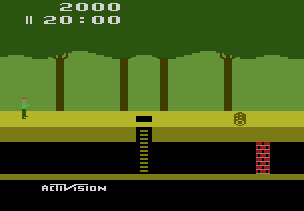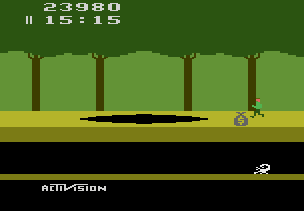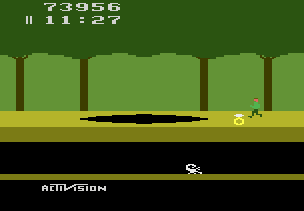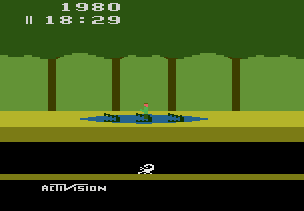# Tutorial #4 – Arithmetic Operations

## Overview

This tutorial will show a few examples of how to create achievements for a game that requires Arithmetic Operations to convert memory values to Base 10 decimal values. Pitfall! was chosen for this example because it has different encoding techniques for the time, score, and lives.## Decimal (Base 10)

There are many ways to describe a number value and we commonly use the decimal system in everyday language. The decimal system is Base 10 which describes how many possible values there are in each place value. In a Base 10 system the ones place value can be the numbers 0 to 9, after 9 you add one to the next place value to get the number 10. Similarly, the tens place value can be the numbers 0 to 9, after 9 you add one to the hundreds place value to get the number 100.

## Binary (Base 2)

Computers think in binary which is a Base 2 number system. Base 2 is a simplified numbering system where each place value is either b1 which means an electrical current was present or b0 which means no current was present (note that the b before the number indicates a binary number). In a Base 2 system the first place value can be the numbers b0 or b1, to represent the number 2 we need the second place value b10. Similarly, the second place value can either be a b1 or a b0, to represent the number 4 we need a third place value b100.

Another common encoding for computers is hexadecimal which is a Base 16 system. The reason why hexadecimal is a popular counting system on computers is that it translates very easily to the binary system. In a Base 16 system there 16 possible numbers per place value which can be represented b0000 to b1111 in binary. In hexadecimal the number 10, 11, 12, 13, 14, and 15 are written as 0xA, 0xB, 0xC, 0xD, 0xE, and 0xF respectively (note that the 0x before the number indicates a hexadecimal number). To represent a number greater than 15 you need two hexadecimal place values, for example the number 16 is 0x10. Similarly, the second place value can be the numbers 0x0 to 0xF, after 0xF you add one to the third place value to get the number 0x100 (or 256 in decimal).

## Binary-Coded Decimal (BCD)

A very common method of encoding values that are displayed on the screen is to use Binary-Coded Decimal (BCD). BCD is a combination of Base 10 and Base 16 systems. BCD uses Base 10 counting in the respect that each place value can be between 0 to 9. However, this value is store as a Base 16 value in memory which means the values above 9 are ignored. The reason for encoding values in BCD is that is simplifies displaying the value on the screen. When drawing a score on the screen the program will look every 4 bits in the score which will be a value between 0 and 9, then the program would draw the sprite for that number and move on to the next 4 bits. Storing values as BCD makes it much easier to display on a screen then storing a value in Base 10 which would require a conversion before displaying.

# Example 4A: Golden Log

Pitfall! Is a platforming game where you help Pitfall Harry traverse 255 horizontally connected screens of jungle avoiding obstacles and dangerous animals along the way. The goal of Pitfall! is to recover the 32 hidden treasures before the 20:00 time limit expires. Each collected treasure increases the score between 2,000 and 5,000 points depending on the treasure type. The player will lose points by touching a log or falling down into a pit. This makes the players score an excellent measure of how a player is preforming.``````// Pitfall!
// #ID = 11191

// \$001E: 00=game active
function GameActive() => byte(0x00001E) == 0

// \$0055: Score XX0000
// \$0056: Score 00XX00
// \$0057: Score 0000XX
function ScoreX00000() => high4(0x000055)
function Score0X0000() => low4(0x000055)
function Score00X000() => high4(0x000056)
function Score000X00() => low4(0x000056)
function Score0000X0() => high4(0x000057)
function Score00000X() => low4(0x000057)

// Converts the BCD score values into Base 10 decimal
function Score()
{
return ScoreX00000() * 100000 +
Score0X0000() * 10000 +
Score00X000() * 1000 +
Score000X00() * 100 +
Score0000X0() * 10 +
Score00000X()
}

// Check if the score goes from between low and high score to above the high score
function ScoreRange(lowScore, highScore)
{
return prev(Score()) > lowScore &&
prev(Score()) < highScore &&
measured(Score() >= highScore)
}

// Create an achievement for scoring more than 25,000 points
achievement(
title = "Example 4A: Golden Log",
description = "Score 25000 points",
points = 0,
trigger =  GameActive() &&
ScoreRange(15000, 25000)
)
``````

## Score()

The Score() function in this example handles the conversion from the displayed BCD value to a decimal value. For clarity, each place value in memory is given a function with the naming convention showing an X over the place value for that memory. The value in hundred thousands place is named ScoreX00000(), the value in the ten thousands place is Score0X0000(), and so on until we get to the ones place value named Score00000X(). To convert these BCD memory values to a decimal value we must multiply each by its place value and sum them together. The result is a number that we can use for achievement comparisons, rich presence text, or leaderboards.

## ScoreRange()

The ScoreRange() function uses the Score() function to convert the previous and current score values from BCD to decimal values. The ScoreRange() function takes * lowScore* and * highScore* parameters then checks if the score goes from between lowScore and highScore to above the highScore. ScoreRange() also incorporates a measure on the current score value so that the user gets feedback on how close they are to achieving the score.

Since this function converts the BCD score to a decimal value we can enter any score value as parameters. The lowScore 15,000 and highScore 25,000 were selected since the maximum frame score you can get in one frame is 5,000 points for collecting a diamond ring. By doubling the maximum frame score to 10,000 points we give ourselves a comfortable test range. If the player is playing legitimately they will never increase more than 10,000 points in a frame so the achievement will trigger when they reach 25,000 points. However, if the player is cheating, loads a save file, or the score memory is initially a large value when the emulator starts up then, the achievement will not trigger. We don’t have to worry about save files for an Atari 2600 game however, the other two issues are a concern.

Scripts: Example #4A script

# Example 4B: Golden Scorpion

The challenge of Pitfall! comes from the strict time limit of needing to collect all 32 treasures in 20 minutes. The time limit is so strict that you need to utilize some of the underground shortcuts to collect all the treasures in time. Traversing an underground passage is equivalent to traversing three screens above ground so you can speed through very quickly underground. However, treasures only appear above ground and some underground passages are dead ends so you must map you route carefully to succeed. The following achievement rewards the player for scoring 70,000 points in under 15 minutes. To accomplish this the player must utilize the underground passages.``````// Pitfall!
// #ID = 11191

// \$001E: 00=game active
function GameActive() => byte(0x00001E) == 0

// \$0055: Score XX0000
// \$0056: Score 00XX00
// \$0057: Score 0000XX
function ScoreX00000() => high4(0x000055)
function Score0X0000() => low4(0x000055)
function Score00X000() => high4(0x000056)
function Score000X00() => low4(0x000056)
function Score0000X0() => high4(0x000057)
function Score00000X() => low4(0x000057)

// Converts the BCD score values into Base 10 decimal
function Score()
{
return ScoreX00000() * 100000 +
Score0X0000() * 10000 +
Score00X000() * 1000 +
Score000X00() * 100 +
Score0000X0() * 10 +
Score00000X()
}

// \$0058: Time XX:00 (BCD)
//        20=out of game
// \$0059: Time 00:XX (BCD)
// \$005A: Time (miliseconds)
function TimeX00000() => high4(0x000058)
function Time0X0000() => low4(0x000058)
function Time00X000() => high4(0x000059)
function Time000X00() => low4(0x000059)
function Time0000XX() => byte(0x00005A)

// Converts the BCD time values into frames
// Note that minutes and seconds are BCD and the frames are already Base 10
function Time()
{
return TimeX00000() * 36000 +
Time0X0000() * 3600 +
Time00X000() * 600 +
Time000X00() * 60 +
Time0000XX()
}

// Convert the time minutes:seconds:frames to frames (1/60s each)
function TimeSpan(minutes, seconds, frames) => minutes * 3600 + seconds * 60 + frames

// Check if the score goes from between low and high score to above the high score
function ScoreRange(lowScore, highScore)
{
return prev(Score()) > lowScore &&
prev(Score()) < highScore &&
measured(Score() >= highScore)
}

// Create an achievement for scoring more than 75,000 points in 15 minutes or less
achievement(
title = "Example 4B: Golden Scorpion",
description = "Score 70000 points in 15 minutes or less",
points = 0,
trigger = GameActive() &&
ScoreRange(60000, 70000) &&
unless(Time() < TimeSpan(5,0,0))
)
``````

## Time()

The Time() function in this example handles the conversion from the displayed BCD time value to a decimal value in frames. It uses a very similar method to convert the BDC value as Score() however, the values used for each place differ since there are 60 frames in a second and 60 seconds in a minute. To get the multiplication values that we multiply each place value by we need to calculate how many frames are in one place value. The easiest way to do it is to start at the lowest place value, one frame, and then figure how many frames are in the next place value.

``````1 Frame = 1 Frame
1 Second = 60 Frames
10 Seconds = 600 Frames
1 minutes = 60 seconds = 3600 Frames
10 minutes = 600 seconds = 36000 Frames
``````

## TimeSpan()

To complement the Time() function we use the TimeSpan() function to figure out how many frames are in a time span. The TimeSpan() function takes the parameters minutes, seconds, and frames then multiplies the hours by 3600 frames, seconds by 60 frames, and adds them to the frames which is already in frames. The code uses this helper function to figure out how many frames there are left when the game clock is 5:00 (ie. 15 minutes after the start of the game). You could use the value 18,000 frames directly however, writing a helper function like this is more convenient and makes the code easier to read.

Scripts: Example #4B script

# Example 4C: Golden Survivor

There are many dangers in the jungle and Pitfall Harry must proceed carefully to stay safe. Harry only has two extra lives before the game is over! Since there is no opportunity to regain lives each life is very valuable.## Homework #4

A) Using the functions in the previous example make an achievement for scoring 50,000 points without dying. Additionally, use memory address \$000000 which encodes the 1st life as bit 5 and the 2nd life as bit 7.

Solutions: Tutorial #4 Solution

All RAScript Tutorials can be found here.